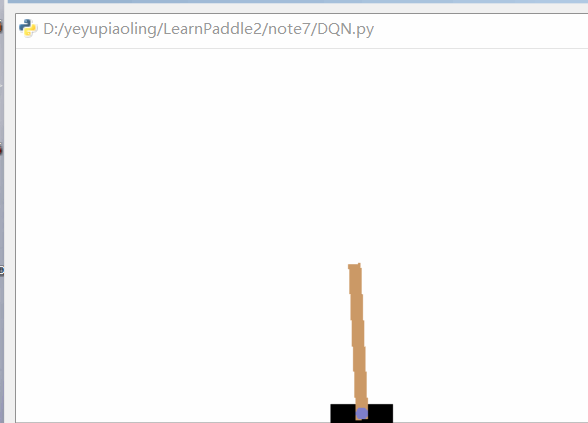# 前言``````import numpy as np
import paddle.fluid as fluid
import random
import gym
from collections import deque
from paddle.fluid.param_attr import ParamAttr
``````

``````# 定义一个深度神经网络，通过指定参数名称，用于之后更新指定的网络参数
def DQNetWork(ipt, variable_field):
fc1 = fluid.layers.fc(input=ipt,
size=24,
act='relu',
param_attr=ParamAttr(name='{}_fc1'.format(variable_field)),
bias_attr=ParamAttr(name='{}_fc1_b'.format(variable_field)))
fc2 = fluid.layers.fc(input=fc1,
size=24,
act='relu',
param_attr=ParamAttr(name='{}_fc2'.format(variable_field)),
bias_attr=ParamAttr(name='{}_fc2_b'.format(variable_field)))
out = fluid.layers.fc(input=fc2,
size=2,
param_attr=ParamAttr(name='{}_fc3'.format(variable_field)),
bias_attr=ParamAttr(name='{}_fc3_b'.format(variable_field)))
return out
``````

``````# 定义更新参数程序
def _build_sync_target_network():
# 获取所有的参数
vars = list(fluid.default_main_program().list_vars())
# 把两个网络的参数分别过滤出来
policy_vars = list(filter(lambda x: 'GRAD' not in x.name and 'policy' in x.name, vars))
target_vars = list(filter(lambda x: 'GRAD' not in x.name and 'target' in x.name, vars))
policy_vars.sort(key=lambda x: x.name)
target_vars.sort(key=lambda x: x.name)

# 从主程序中克隆一个程序用于更新参数
sync_program = fluid.default_main_program().clone()
with fluid.program_guard(sync_program):
sync_ops = []
for i, var in enumerate(policy_vars):
sync_op = fluid.layers.assign(policy_vars[i], target_vars[i])
sync_ops.append(sync_op)
# 修剪第二个玩了个的参数，完成更新参数
sync_program = sync_program._prune(sync_ops)
return sync_program
``````

``````# 定义输入数据
state_data = fluid.layers.data(name='state', shape=, dtype='float32')
action_data = fluid.layers.data(name='action', shape=, dtype='int64')
reward_data = fluid.layers.data(name='reward', shape=[], dtype='float32')
next_state_data = fluid.layers.data(name='next_state', shape=, dtype='float32')
done_data = fluid.layers.data(name='done', shape=[], dtype='float32')
``````

``````# 定义训练的参数
batch_size = 32
num_episodes = 300
num_exploration_episodes = 100
max_len_episode = 1000
learning_rate = 1e-3
gamma = 1.0
initial_epsilon = 1.0
final_epsilon = 0.01
``````

``````# 实例化一个游戏环境，参数为游戏名称
env = gym.make("CartPole-v1")
replay_buffer = deque(maxlen=10000)
``````

``````# 获取网络
state_model = DQNetWork(state_data, 'policy')
``````

``````# 克隆预测程序
predict_program = fluid.default_main_program().clone()
``````

``````# 定义损失函数
action_onehot = fluid.layers.one_hot(action_data, 2)
action_value = fluid.layers.elementwise_mul(action_onehot, state_model)
pred_action_value = fluid.layers.reduce_sum(action_value, dim=1)

targetQ_predict_value = DQNetWork(next_state_data, 'target')
best_v = fluid.layers.reduce_max(targetQ_predict_value, dim=1)
target = reward_data + gamma * best_v * (1.0 - done_data)

cost = fluid.layers.square_error_cost(pred_action_value, target)
avg_cost = fluid.layers.reduce_mean(cost)
``````

``````# 获取更新参数程序
_sync_program = _build_sync_target_network()
``````

``````# 定义优化方法
optimizer = fluid.optimizer.AdamOptimizer(learning_rate=learning_rate, epsilon=1e-3)
opt = optimizer.minimize(avg_cost)
``````

``````# 创建执行器并进行初始化
place = fluid.CPUPlace()
exe = fluid.Executor(place)
exe.run(fluid.default_startup_program())
epsilon = initial_epsilon
``````

• 在每次循环开始，就开始获取游戏的状态，这个是游戏结束之后再执行的。
• 定义一个 epsilon-greedy 探索策略，这个策略是根据训练的进度，开始选择自动操作的动作或者是模型预测的动作的概率。
• 接下来就是一局游戏的的循环，在这里可以显示游戏的界面
• 下面就是通过使用 epsilon-greedy 探索策略，决定使用随机生成动作，还是预测生成动作，使用随机动作可以增加数据的多样性，通过使用模型预测就是让模型根据当前的游戏状态来预测下一步动作是怎么才是正确的，随着模型的不断训练，这个预测也是越来越正确。
• 然后更加随机生成的动作，或者模型预测的动作，传递个游戏，得到游戏的相关输出，比如游戏的下一个状态，游戏的奖励，游戏是否结束等等。
• 如果游戏结束了，应当给游戏一个负奖励，惩罚它做出了一个错误的操作。
• 然后把这些数据存储起来，用于之后训练使用。
• 当存储的数据大于或等于 Batch size，就可以开始训练。
``````update_num = 0
# 开始玩游戏
for epsilon_id in range(num_episodes):
# 初始化环境，获得初始状态
state = env.reset()
epsilon = max(initial_epsilon * (num_exploration_episodes - epsilon_id) /
num_exploration_episodes, final_epsilon)
for t in range(max_len_episode):
# 显示游戏界面
# env.render()
state = np.expand_dims(state, axis=0)
# epsilon-greedy 探索策略
if random.random() < epsilon:
# 以 epsilon 的概率选择随机下一步动作
action = env.action_space.sample()
else:
# 使用模型预测作为结果下一步动作
action = exe.run(predict_program,
feed={'state': state.astype('float32')},
fetch_list=[state_model])
action = np.squeeze(action, axis=0)
action = np.argmax(action)

# 让游戏执行动作，获得执行完 动作的下一个状态，动作的奖励，游戏是否已结束以及额外信息
next_state, reward, done, info = env.step(action)

# 如果游戏结束，就进行惩罚
reward = -10 if done else reward
# 记录游戏输出的结果，作为之后训练的数据
replay_buffer.append((state, action, reward, next_state, done))
state = next_state

# 如果游戏结束，就重新玩游戏
if done:
print('Pass:%d, epsilon:%f, score:%d' % (epsilon_id, epsilon, t))
break

# 如果收集的数据大于Batch的大小，就开始训练
if len(replay_buffer) >= batch_size:
batch_state, batch_action, batch_reward, batch_next_state, batch_done = \
[np.array(a, np.float32) for a in zip(*random.sample(replay_buffer, batch_size))]

# 更新参数
if update_num % 200 == 0:
exe.run(program=_sync_program)
update_num += 1

# 调整数据维度
batch_action = np.expand_dims(batch_action, axis=-1)
batch_next_state = np.expand_dims(batch_next_state, axis=1)

# 执行训练
exe.run(program=fluid.default_main_program(),
feed={'state': batch_state,
'action': batch_action.astype('int64'),
'reward': batch_reward,
'next_state': batch_next_state,
'done': batch_done})
``````

``````......
Pass:70, epsilon:0.300000, score:234
Pass:71, epsilon:0.290000, score:272
Pass:72, epsilon:0.280000, score:254
Pass:73, epsilon:0.270000, score:148
Pass:74, epsilon:0.260000, score:147
Pass:75, epsilon:0.250000, score:342
Pass:76, epsilon:0.240000, score:295
Pass:77, epsilon:0.230000, score:290
Pass:78, epsilon:0.220000, score:276
Pass:79, epsilon:0.210000, score:279
......
``````